Worksheets and No Prep Teaching Resources Math Worksheets Learning Centers Math Learning Centers

Telling Time Math Center (easier - hour hand does not move between numbers)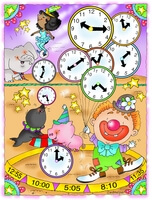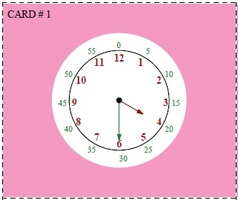Minutes shown on clock (Grades K-1) Normal clock - just hours shown on clock (Grades 1-3)

Telling Time Math Center (more difficult - hour hand moves with minutes like a real clock)Minutes shown on clock (Grades K-1) Normal clock - just hours shown on clock (Grades 1-3)

Addition Math Center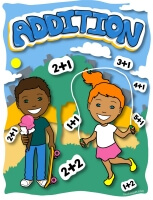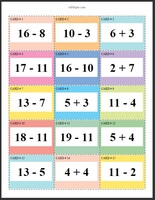Addition Math Center: Sums of 2 to 9 (Grades 3-6) Addition Math Center: Sums of 2 to 19 (Grades 3-6)

Addition and Subtraction Math Center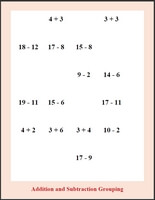Sums and Differences of 2 to 9 (Grades 3-6) Sums and Differences of 2 to 19 (Grades 3-6)

Addition Stairs Math Learning Center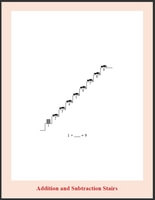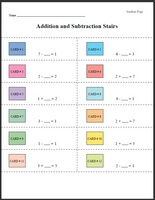Addition Stairs: Numbers 1 to 5 (Grades 1-3) Addition Stairs: Numbers 1 to 9 (Grades 1-3) Addition Stairs: Numbers 1 to 14 (Grades 1-3) Addition Stairs: Numbers 1 to 19 (Grades 1-3)

Subtraction Math Center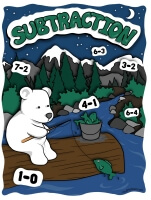Subtraction Math Center: Differences of 2 to 9 (Grades 3-6) Subtraction Math Center: Differences of 2 to 19 (Grades 3-6)

Subtraction Stairs Math Learning CenterSubtraction Stairs: Numbers 1 to 5 (Grades 1-3) Subtraction Stairs: Numbers 1 to 9 (Grades 1-3) Subtraction Stairs: Numbers 1 to 14 (Grades 1-3) Subtraction Stairs: Numbers 1 to 19 (Grades 1-3)

Multiplication Math CenterMultiplication Math Center: Numbers 2 to 9 - Products of 2 to 81 (Grades 3-6) Multiplication Math Center: Numbers 2 to 12 - Products of 2 to 81 (Grades 3-6)

Multiplication and Division Math CenterMultiplication and Division Math Center: Numbers 2 to 9 (Grades 3-6) Multiplication and Division Math Center: Numbers 2 to 12 (Grades 3-6)

Division Math CenterDivision Math Center: Numbers 2 to 9 (Grades 3-6) Division Math Center: Numbers 2 to 12 (Grades 3-6)

Fractions Math Center - Write the Fraction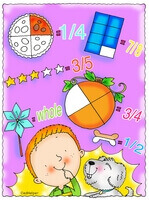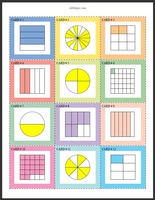Write the fraction - numerator is always 1 (Grades 2-4) Write the fraction (Grades 3-6) Write the fraction - a blank on student page (student will need to know to draw line and where to put numerator and denominator) (Grades 3-6)

Matching - Picture of Fractions and Fractions - Math Learning Center

Matching - Picture of Fractions, Fractions, and Decimals - Math Learning Center

Matching - Fractions and Decimals - Math Learning Center

Place Value Math Center - Ones, Tens, and Hundreds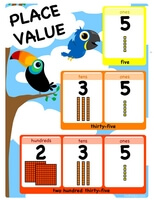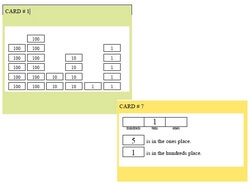Easier: Place value words are given under boxes (first answer given) (Grades 1-3) Easier: Place value words are given under boxes (Grades 1-3) Ones, tens, and hundreds place value math learning center (first answer given) (Grades 1-3) Ones, tens, and hundreds place value math learning center (Grades 1-3) Easier: Place value words are given under boxes (first answer given) (Grades 1-3)

Place Value Math Center - Ones, Tens, Hundreds, and ThousandsEasier: Place value words are given under boxes (first answer given) (Grades 1-3) Easier: Place value words are given under boxes (Grades 1-3) Ones, tens, hundreds, and thousands place value math learning center (first answer given) (Grades 1-3) Ones, tens, hundreds, and thousands place value math learning center (Grades 1-3)

Place Value Math Center - Ones, Tens, Hundreds, Thousands, and Ten-ThousandsEasier: Place value words are given under boxes (first answer given) (Grades 1-3) Easier: Place value words are given under boxes (Grades 1-3) Ones, tens, hundreds, thousands, and ten-thousands place value math learning center (first answer given) (Grades 1-3) Ones, tens, hundreds, thousands, and ten-thousands place value math learning center (Grades 1-3)

Plotting Ordered Pairs to Make a Picture Math Center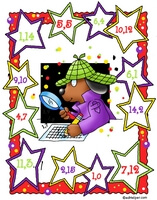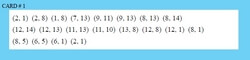Plotting Ordered Pairs - smaller coordinate plane (Grades 1-4) Plotting Ordered Pairs - smaller coordinate plane - student will also write what they think the picture is (Grades 1-4) Plotting Ordered Pairs - larger coordinate plane (Grades 4-6) Plotting Ordered Pairs - larger coordinate plane - student will also write what they think the picture is (Grades 4-6)

Math Learning Center: Counting Coins and Money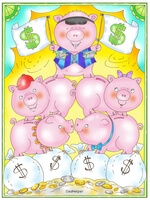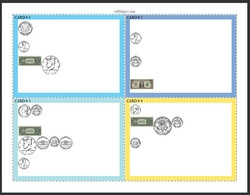U.S. Money: Coins only (Grades 3-6) U.S. Money: Bills only (Grades 3-6) U.S. Money: At least one coin and one bill on each card (Grades 3-6) U.S. Money: Mix (Grades 3-6)

Euros - Math Learning Center: Counting Coins and MoneyEuros: Coins less than 100 Euros (Grades 3-6) Euros: Coins at least 100 Eurus (Grades 3-6) Euros: Mix of small and large coins (Grades 3-6) Euros: Mix (Grades 3-6)

Canadian Currency - Math Learning Center: Counting Coins and MoneyCanadian Currency: Coins less than 100 cents (Grades 3-6) Canadian Currency: Coins at least 100 cents (Grades 3-6) Canadian Currency: Mix of small and large coins (Grades 3-6) Canadian Currency: Mix (Grades 3-6)

Color Gumballs Math Learning Center: Estimate - Match Picture of Gumballs with Estimate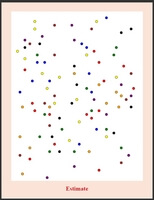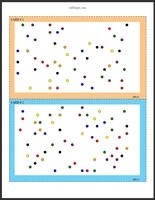Gumballs: Estimate by tens - 10, 20, up to 100 - Ten Cards - Match Estimate with Picture (Grades 2-6) Gumballs: Estimate by tens - Five Cards (Grades 2-6)

Black and White Rectangles to Estimate - Math Learning Center: Match Picture with EstimateEstimate by tens - 10, 20, up to 100 - Ten Cards - Match Estimate with Picture (Grades 2-6) Estimate by tens - Five Cards (Grades 2-6)

Writing an Estimate - Math Learning CenterTens: Writing an Estimate - Ten Cards (Grades 4-6) Tens: Writing an Estimate - Five Cards (Grades 4-6)

Color Gumballs Math Learning Center: Estimate - Match Picture of Gumballs with EstimateGumballs: Estimate by tens - 10, 20, up to 100 - Ten Cards - Match Estimate with Picture (Grades 2-6) Gumballs: Estimate by tens - Five Cards (Grades 2-6)

Black and White Rectangles to Estimate - Math Learning Center: Match Picture with EstimateEstimate by tens - 10, 20, up to 100 - Ten Cards - Match Estimate with Picture (Grades 2-6) Estimate by tens - Five Cards (Grades 2-6)

Writing an Estimate - Math Learning CenterTens: Writing an Estimate - Ten Cards (Grades 4-6) Tens: Writing an Estimate - Five Cards (Grades 4-6)

Easiest (horizontal patterns only): Complete the Pattern Math Learning Centers - Large Fonts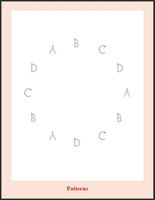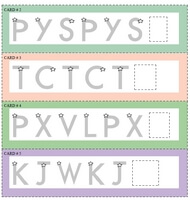easy patterns, just two letters repeating: ABABABAB (Grades 2-6) easy patterns, two to four different letters repeating (Grades 2-6) easy patterns and also some three letter patterns with one letter repeating (Grades 2-6) easy patterns and also some three or four letter patterns with letters repeating (Grades 2-6)

Complete the Pattern Math Learning Centers - Large Fontseasy patterns, just two letters repeating: ABABABAB (Grades 2-6) easy patterns, two to four different letters repeating (Grades 2-6) easy patterns and also some three letter patterns with one letter repeating (Grades 2-6) easy patterns and also some three or four letter patterns with letters repeating (Grades 2-6)

Complete the Pattern Math Learning Centers - Smaller Fontseasy patterns, just two letters repeating: ABABABAB (Grades 2-6) easy patterns, two to four different letters repeating (Grades 2-6) easy patterns and also some three letter patterns with one letter repeating (Grades 2-6) easy patterns and also some three or four letter patterns with letters repeating (Grades 2-6)

Complete the Pattern Math Learning Centers - Picture Patternseasy patterns, just two pictures repeating (Grades 2-6) easy patterns, two to four different pictures repeating (Grades 2-6) easy patterns and also some three picture patterns with one picture repeating (Grades 2-6) easy patterns and also some three or four picture patterns with pictures repeating (Grades 2-6)

Complete the Pattern Math Learning Centers - Some Picture Patterns and Some Letter Patternseasy patterns, just two pictures or letters repeating (Grades 2-6) easy patterns, two to four different pictures or letters repeating (Grades 2-6) easy patterns and also some three picture or letter patterns with repeating in pattern (Grades 2-6) easy patterns and also some three or four picture or letter patterns with repeating in pattern (Grades 2-6)

Mean, Median, Mode, and Range Math Center - Answering Questions

Mean, Median, Mode, and Range Math Center - Order the Numbers and Answer Questions

Ordering Numbers Math Center - Whole Numbers

Ordering Numbers Math Center - Fractions

Ordering Numbers Math Center - Negative Numbers

Ordering Numbers Math Center - Decimals

Addition and Subtraction Stairs Math Learning CenterAddition and Subtraction Stairs: Numbers 1 to 5 (Grades 1-3) Addition and Subtraction Stairs: Numbers 1 to 9 (Grades 1-3) Addition and Subtraction Stairs: Numbers 1 to 14 (Grades 1-3) Addition and Subtraction Stairs: Numbers 1 to 19 (Grades 1-3)

Complete Hundreds Chart (1 to 100) Math Learning Center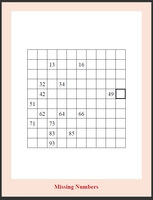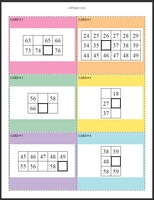(complete chart on each card) Easiest (Grades 1-3) (complete chart on each card) A few more blanks: Write one of the missing numbers (Grades 1-3) (complete chart on each card) Most Difficult: Numbers not next to blank (Grades 1-3)

Numbers Chart Math Learning Center - 5 rows on each card(5 rows on each card) Easiest (Grades 1-3) (5 rows on each card) A few numbers not shown (Grades 1-3) (5 rows on each card) More Difficult: will have blank boxes next to the number that needs to be written (Grades 1-3) (5 rows on each card) Only one number is on each chart - number can be in the same row as the number to write (Grades 1-3) (5 rows on each card) Most Difficult: Only one number is on each chart and number cannot be in the same row as the number to write (Grades 1-3)

Numbers Chart Math Learning Center - 3 to 4 rows on each card(3 to 4 rows on each card) Easiest (Grades 1-3) (3 to 4 rows on each card) A few numbers not shown (Grades 1-3) (3 to 4 rows on each card) More Difficult: will have blank boxes next to the number that needs to be written (Grades 1-3) (3 to 4 rows on each card) Only one number is on each chart - number can be in the same row as the number to write (Grades 1-3) (3 to 4 rows on each card) Most Difficult: Only one number is on each chart and number cannot be in the same row as the number to write (Grades 1-3)

Numbers Chart Math Learning Center - 2 to 3 rows on each card(2 to 3 rows on each card) Easiest (Grades 1-3) (2 to 3 rows on each card) A few numbers not shown (Grades 1-3) (2 to 3 rows on each card) More Difficult: will have blank boxes next to the number that needs to be written (Grades 1-3) (2 to 3 rows on each card) Only one number is on each chart - number can be in the same row as the number to write (Grades 1-3) (2 to 3 rows on each card) Most Difficult: Only one number is on each chart and number cannot be in the same row as the number to write (Grades 1-3)

Easiest: Measurement Math Center - Inches - Fewer Images on Cards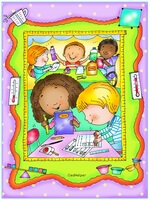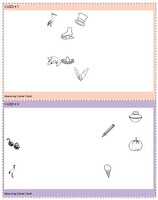Easiest: Measure the length between two pictures - Horizontal Measure Only (full page cards) (Grades 1-4) Easiest: Measure the length between two pictures - Horizontal Measure Only (half-page cards) (Grades 1-4) Easiest: Measure the length between two pictures - Vertical Measure Only (full page cards) (Grades 1-4) Easiest: Measure the length between two pictures - Vertical Measure Only (half-page cards) (Grades 1-4) Measure the length between two pictures - Horizontal or Vertical Measure (full page cards) (Grades 1-4) Measure the length between two pictures - Horizontal or Vertical Measure (half-page cards) (Grades 1-4) Measure the length between two pictures (full page cards) (Grades 1-4) Measure the length between two pictures (half-page cards) (Grades 1-4)

Measurement Math Center - InchesMeasure the length between two pictures (full page cards) (Grades 1-4) Measure the length between two pictures (half-page cards) (Grades 1-4)

Easiest: Measurement Math Center - Centimeters - Fewer Images on CardsEasiest: Measure the length between two pictures - Horizontal Measure Only (full page cards) (Grades 1-4) Easiest: Measure the length between two pictures - Horizontal Measure Only (half-page cards) (Grades 1-4) Easiest: Measure the length between two pictures - Vertical Measure Only (full page cards) (Grades 1-4) Easiest: Measure the length between two pictures - Vertical Measure Only (half-page cards) (Grades 1-4) Measure the length between two pictures - Horizontal or Vertical Measure (full page cards) (Grades 1-4) Measure the length between two pictures - Horizontal or Vertical Measure (half-page cards) (Grades 1-4) Measure the length between two pictures (full page cards) (Grades 1-4) Measure the length between two pictures (half-page cards) (Grades 1-4)

Measurement Math Center - CentimetersMeasure the length between two pictures (full page cards) (Grades 1-4) Measure the length between two pictures (half-page cards) (Grades 1-4)

Count By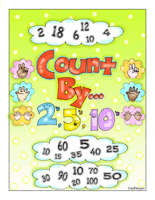Count By... 2s, 5s, 10s

Odd and Even Activity Book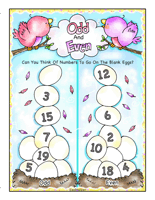Odd And Even

Locker Combo Math Learning Center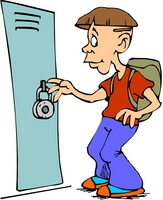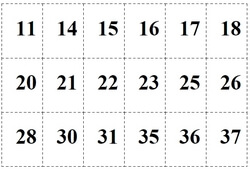Easiest: Open the Locker - Only 1 Number (Grades 4-5) Open the Locker - 2 Numbers in the Locker Combo (Grades 5-6) Open the Locker - 3 Numbers in the Locker Combo (Grades 5-6)

Have a suggestion or would like to leave feedback?
Leave your suggestions or comments about edHelper!